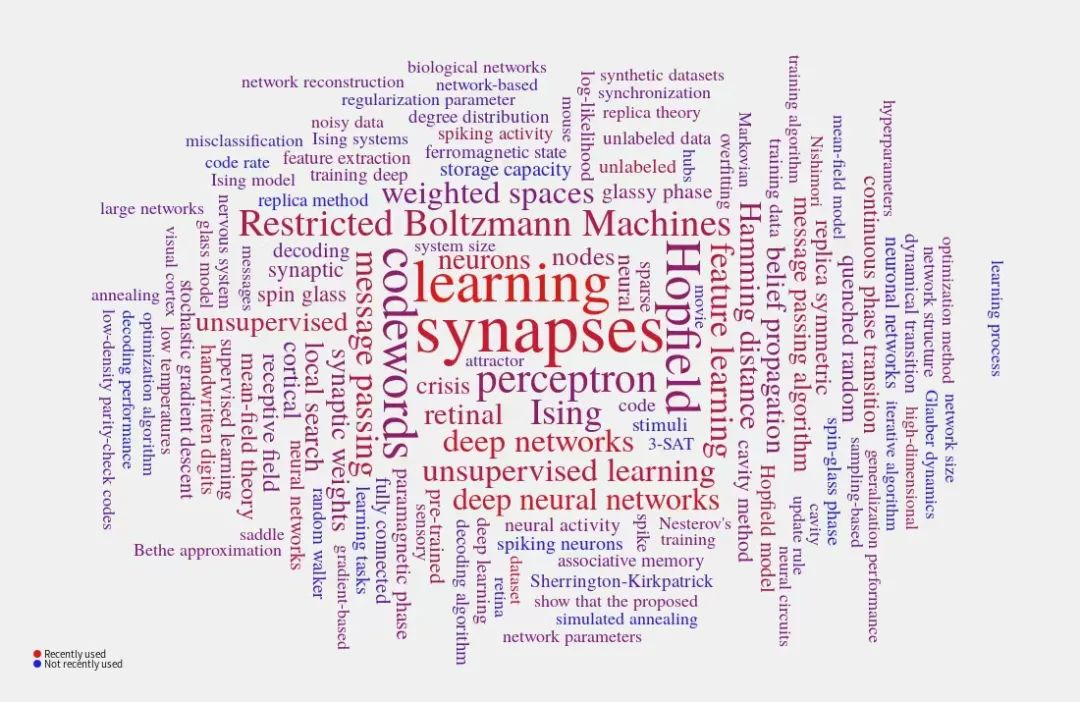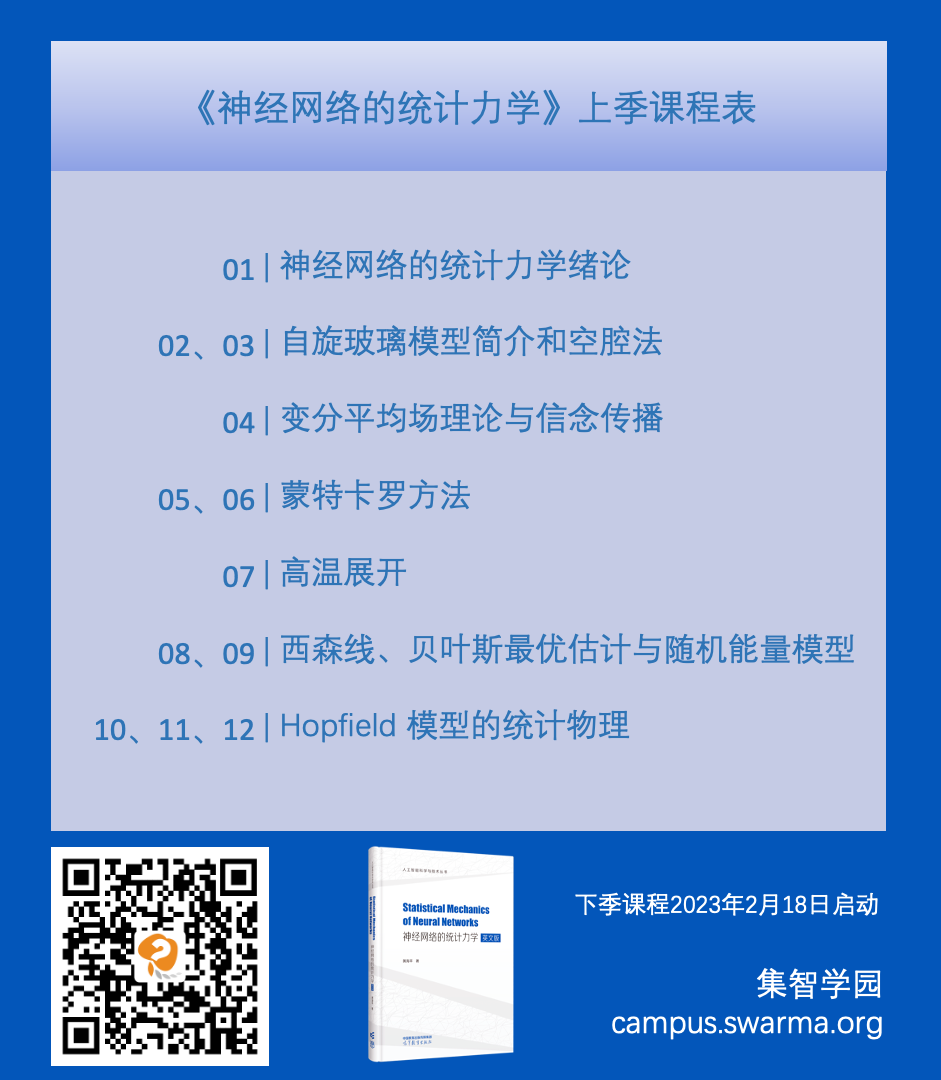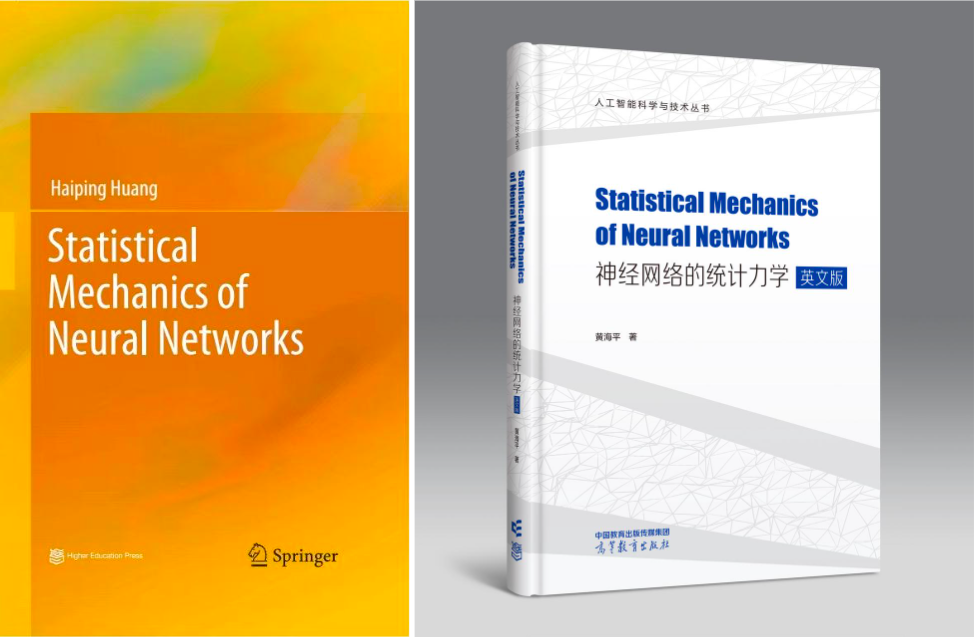### 负责人介绍PMI Lab：https://www.labxing.com/hphuang2018

### 本季课程回顾Chapter 1:  Introduction

Chapter 2:  Spin Glass Models and Cavity Method

• 2.1 Multi-spin Interaction Models
• 2.2 Cavity Method
• 2.3 From Cavity Method to Message Passing Algorithms

Chapter 3:  Variational Mean-Field Theory and Belief Propagation

• 3.1 Variational Method
• 3.2 Variational Free Energy
• 3.3 Mean-Field Inverse Ising Problem

Chapter 4:  Monte Carlo Simulation Methods

• 4.1 Monte Carlo Method
• 4.2 Importance Sampling
• 4.3 Markov Chain Sampling
• 4.4 Monte Carlo Simulations in Statistical Physics

Chapter 5:  High-Temperature Expansion

• 5.1 Statistical Physics Setting
• 5.2 High-Temperature Expansion
• 5.3 Properties of the TAP Equation

Chapter 6:  Nishimori Line

• 6.1 Model Setting
• 6.2 Exact Result for Internal Energy
• 6.3 Proof of No RSB Effects on the Nishimori Line

Chapter 7:  Random Energy Model

• 7.1 Model Setting
• 7.2 Phase Diagram

Chapter 8:  Statistical Mechanical Theory of Hopfifield Model

• 8.1 Hopfifield Model
• 8.2 Replica Method
• 8.3 Phase Diagram
• 8.4 Hopfifield Model with Arbitrary Hebbian Length

### 下季内容预告

Chapter 9:  Replica Symmetry and Replica Symmetry Breaking

• 9.1 Generalized Free Energy and Complexity of States
• 9.2 Applications to Constraint Satisfaction Problems
• 9.3 More Steps of Replica Symmetry Breaking

Chapter 10:  Statistical Mechanics of Restricted Boltzmann Machine

• 10.1 Boltzmann Machine
• 10.2 Restricted Boltzmann Machine
• 10.3 Free Energy Calculation
• 10.4 Thermodynamic Quantities Related to Learning
• 10.5 Stability Analysis
• 10.6 Variational Mean-Field Theory for Training Binary RBMs

Chapter 11:  Simplest Model of Unsupervised Learning with Binary

• 11.1 Model Setting
• 11.2 Derivation of sMP and AMP Equations
• 11.3 Replica Computation
• 11.4 Phase Transitions
• 11.5 Measuring the Temperature of Dataset

Chapter 12:  Inherent-Symmetry Breaking in Unsupervised Learning

• 12.1 Model Setting
• 12.2 Phase Diagram
• 12.3 Hyper-Parameters Inference

Chapter 13:  Mean-Field Theory of Ising Perceptron

• 13.1 Ising Perceptron model
• 13.2 Message-Passing-Based Learning
• 13.3 Replica Analysis

Chapter 14:  Mean-Field Model of Multi-layered Perceptron

• 14.1 Random Active Path Model
• 14.2 Mean-Field Training Algorithms
• 14.3 Spike and Slab Model

Chapter 15:  Mean-Field Theory of Dimension Reduction in Neural Networks

• 15.1 Mean-Field Model
• 15.2 Linear Dimensionality and Correlation Strength
• 15.3 Dimension Reduction with Correlated Synapses

Chapter 16:  Chaos Theory of Random Recurrent Neural Networks

• 16.1 Spiking and Rate Models
• 16.2 Dynamical Mean-Field Theory
• 16.3 Lyapunov Exponent and Chaos
• 16.4 Excitation-Inhibition Balance Theory
• 16.5 Training Recurrent Neural Networks

Chapter 17:  Statistical Mechanics of Random Matrices

• 17.1 Spectral Density
• 17.2 Replica Method and Semi-circle Law
• 17.3 Cavity Approach and Marchenko
• 17.4 Spectral Densities of Random Asymmetric Matrices

### 课程书籍介绍Statistical Mechanics of Neural Networks

### 报名加入课程